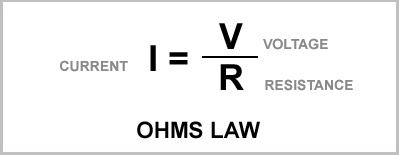## Monday, March 2, 2009

### Fender Champion 600 Preamp Bias Part 2a

The the last post we found one of two points we need to draw the load line for first 12AX7 gain stage in a Champion 600. We'll use the load line to find the bias point and later reset that bias point for the 12DW7 mod.

The first point was found by simply plotting the B+ voltage on the X axis of the 12AX7 plate curves:Now we need to find the maximum plate current. To do this we use Ohm's Law to calculate how much current will flow with the full B+ voltage dropped across the plate resistor.We know the B+ is 340 volts and the plate resistor is 100,000 ohms.

340 divided by 100,000 is .0034 amps or 3.4 milliamps

Now we plot 3.4 mA onto the Y axis and connect that dot to the one we made on the X axis:The line connecting these two points is what's called a "load line" and it's what we use to determine the bias point for the tube. I'll cover the bias point in an upcoming post.